# plotPermutationResults

Plot histogram of permutation results for a variable specified for data drift detection

Since R2022a

## Syntax

``plotPermutationResults(DDiagnostics)``
``plotPermutationResults(DDiagnostics,Variable=variable)``
``plotPermutationResults(ax,___)``
``H = plotPermutationResults(___)``
``[H,CL] = plotPermutationResults(___)``

## Description

example

````plotPermutationResults(DDiagnostics)` plots the histogram of metric values computed by the `driftdetect` function during permutation testing for the variable with the lowest p-value.If you set the value of `EstimatePValues` to `false` in the call to `detectdrift`, then `plotPermutationResults` does not generate a plot and, instead, returns a warning.```

example

````plotPermutationResults(DDiagnostics,Variable=variable)` plots the histogram for the variable specified by `variable`.```

example

````plotPermutationResults(ax,___)` plots on the axes `ax` instead of `gca` using any of the previous input argument combinations in the previous syntaxes.```
````H = plotPermutationResults(___)` plots the histogram and returns an array of `Histogram` objects `H` for the metric values computed during permutation testing. Use `H` to inspect and modify the properties of the histogram. For more information, see Histogram Properties.```

example

````[H,CL] = plotPermutationResults(___)` additionally returns a `ConstantLine` object `CL` for the metric threshold value. Use `CL` to inspect and modify the properties of the line. For more information, see ConstantLine Properties.```

## Examples

collapse all

Generate baseline and target data with three variables, where the distribution parameters of the second and third variables change for the target data.

```rng('default') % For reproducibility baseline = [normrnd(0,1,100,1),wblrnd(1.1,1,100,1),betarnd(1,2,100,1)]; target = [normrnd(0,1,100,1),wblrnd(1.2,2,100,1),betarnd(1.7,2.8,100,1)];```

Perform permutation testing for all variables to check for any drift between the baseline and target data.

`DDiagnostics = detectdrift(baseline,target)`
```DDiagnostics = DriftDiagnostics VariableNames: ["x1" "x2" "x3"] CategoricalVariables: [] DriftStatus: ["Stable" "Drift" "Warning"] PValues: [0.3850 0.0050 0.0910] ConfidenceIntervals: [2×3 double] MultipleTestDriftStatus: "Drift" DriftThreshold: 0.0500 WarningThreshold: 0.1000 Properties, Methods ```

Plot the permutation results for the default variable.

`plotPermutationResults(DDiagnostics)`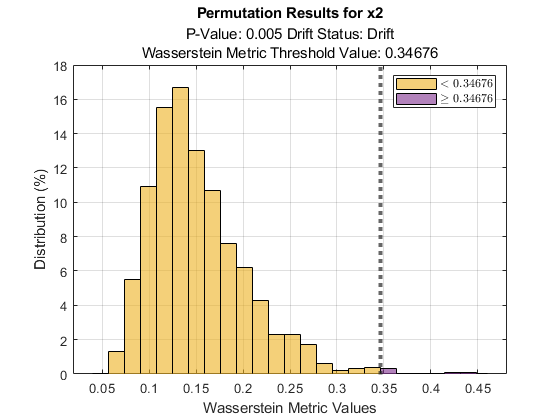By default, `plotPermutationResults` plots a histogram of the metric values computed in permutation testing for the variable with the lowest p-value, which is `x2` in this case. The function includes the metric threshold value (the initial metric value computed by `detectdrift` using the baseline and target data) on the histogram, so you can see the values that are greater than or equal to the threshold. `plotPermutationResults` also displays the p-value and the drift status for the variable, and the metric that you specify to use for permutation testing in the call to `detectdrift`. In this example, no metric is specified, so `detectdrift` uses the default metric (Wasserstein) for continuous variables.

Generate baseline and target data with three variables, where the distribution parameters of the second and third variables change for the target data.

```rng('default') % For reproducibility baseline = [normrnd(0,1,100,1),wblrnd(1.1,1,100,1),betarnd(1,2,100,1)]; target = [normrnd(0,1,100,1),wblrnd(1.2,2,100,1),betarnd(1.7,2.8,100,1)];```

Perform permutation testing for all variables to check for any drift between the baseline and target data. Use the Energy metric for all variables.

`DDiagnostics = detectdrift(baseline,target,ContinuousMetric="energy")`
```DDiagnostics = DriftDiagnostics VariableNames: ["x1" "x2" "x3"] CategoricalVariables: [] DriftStatus: ["Stable" "Drift" "Warning"] PValues: [0.3790 0.0110 0.0820] ConfidenceIntervals: [2×3 double] MultipleTestDriftStatus: "Drift" DriftThreshold: 0.0500 WarningThreshold: 0.1000 Properties, Methods ```

Display the 95% confidence bounds for the p-values.

`DDiagnostics.ConfidenceIntervals`
```ans = 2×3 0.3488 0.0055 0.0657 0.4099 0.0196 0.1008 ```

Plot the permutation results for the third variable.

`plotPermutationResults(DDiagnostics,Variable=3)`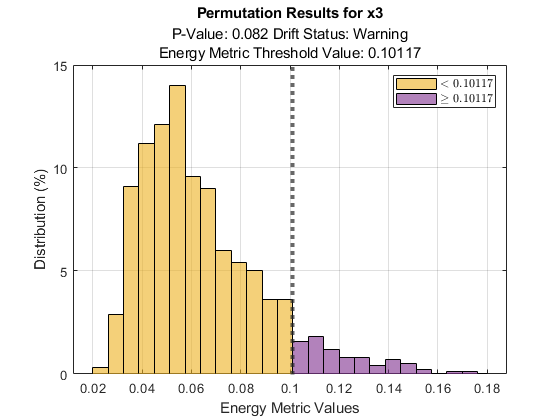Generate baseline and target data with three variables, where the distribution parameters of the second and third variables change for the target data.

```rng('default') % For reproducibility baseline = [normrnd(0,1,100,1),wblrnd(1.1,1,100,1),betarnd(1,2,100,1)]; target = [normrnd(0,1,100,1),wblrnd(1.2,2,100,1),betarnd(1.7,2.8,100,1)];```

Perform permutation testing for all variables to check for any drift between the baseline and target data. Use the Energy metric for all variables.

`DDiagnostics = detectdrift(baseline,target,ContinuousMetric="energy")`
```DDiagnostics = DriftDiagnostics VariableNames: ["x1" "x2" "x3"] CategoricalVariables: [] DriftStatus: ["Stable" "Drift" "Warning"] PValues: [0.3790 0.0110 0.0820] ConfidenceIntervals: [2x3 double] MultipleTestDriftStatus: "Drift" DriftThreshold: 0.0500 WarningThreshold: 0.1000 Properties, Methods ```

Plot the permutation results for variables `x1` and `x2` in a tiled layout.

```tiledlayout(2,1); ax1 = nexttile; plotPermutationResults(DDiagnostics,ax1,Variable="x1") ax2 = nexttile; plotPermutationResults(DDiagnostics,ax2,Variable="x2")```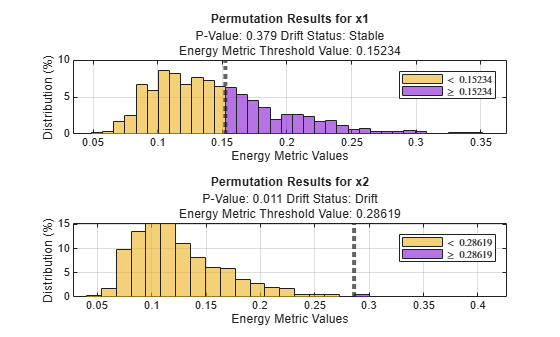Plot the permutation results for variables `x1` and `x3` in a tiled layout.

```tiledlayout(2,1); ax1 = nexttile; plotPermutationResults(DDiagnostics,ax1,Variable="x1") ax3= nexttile; plotPermutationResults(DDiagnostics,ax3,Variable="x3")```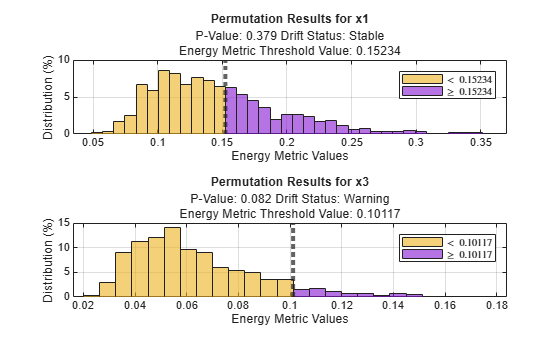Generate baseline and target data with three variables, where the distribution parameters of the second and third variables change for the target data.

```rng('default') % For reproducibility baseline = [normrnd(0,1,100,1),wblrnd(1.1,1,100,1),betarnd(1,2,100,1)]; target = [normrnd(0,1,100,1),wblrnd(1.2,2,100,1),betarnd(1.7,2.8,100,1)];```

Perform permutation testing for all variables to check for any drift between the baseline and target data. Use the Energy distance as the metric.

`DDiagnostics = detectdrift(baseline,target,ContinuousMetric="energy")`
```DDiagnostics = DriftDiagnostics VariableNames: ["x1" "x2" "x3"] CategoricalVariables: [] DriftStatus: ["Stable" "Drift" "Warning"] PValues: [0.3790 0.0110 0.0820] ConfidenceIntervals: [2×3 double] MultipleTestDriftStatus: "Drift" DriftThreshold: 0.0500 WarningThreshold: 0.1000 Properties, Methods ```

Plot the permutation results for the third variable.

`[H,CL] = plotPermutationResults(DDiagnostics,Variable=3)````H = 2×1 Histogram array: Histogram Histogram ```
```CL = ConstantLine with properties: InterceptAxis: 'x' Value: 0.1012 Color: [0.1500 0.1500 0.1500] LineStyle: ':' LineWidth: 3 Label: '' DisplayName: '' Show all properties ```

Change the histogram bar colors to blue and the threshold line color to red.

```H(1).FaceColor = "b"; CL.Color = "r";```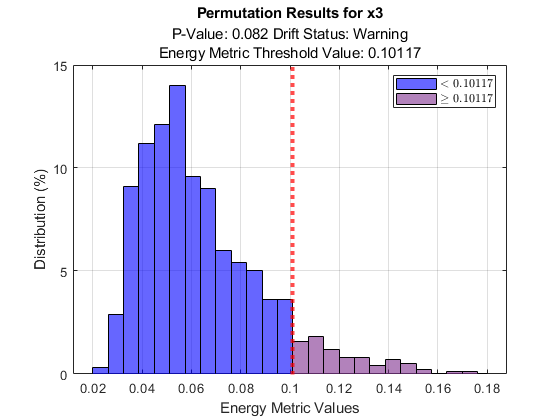You can also access and modify properties by double-clicking `H` or `CL` in the `Workspace` to open and use the `Property Inspector`.

## Input Arguments

collapse all

Diagnostics of the permutation testing for drift detection, specified as a `DriftDiagnostics` object returned by `detectdrift`.

Variable for which to plot the permutation results, specified as a string, character vector, or integer index.

Example: `Variable="x2"`

Example: `Variable=2`

Data Types: `single` | `double` | `char` | `string`

Axes on which to plot, specified as an `Axes` or `UIAxes` object. If you do not specify `ax`, then `plotPermutationResults` creates the plot using the current axes. For more information on creating an axes object, see `axes` and `uiaxes`.

## Output Arguments

collapse all

Histogram of metric values computed during permutation testing, returned as a 2-by-1 array of `Histogram` objects. Use `H` to inspect and adjust the properties of the histogram. For more information on the `Histogram` object properties, see Histogram Properties.

Line showing the metric threshold value in the plot, returned as a `ConstantLine` object. Use `CL` to inspect and modify the properties of the line.

## Version History

Introduced in R2022a